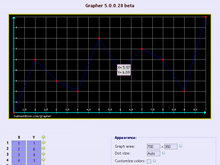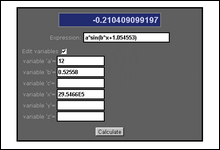Mathematical Tools

In this category you will find tools related to Mathematics. These tools would help you in mathematical calculation and analysis.

Tools under this categoryGrapher is a online graph Plotting tool, it can plot a given set of (x,y) coordinates or any fuction of 'x'. The program can be fully customized to change colors, text size, etc. It has lots of features which helps in easy analysis of Graphs.This program can evaluate / calculate any given expression or function. Variables can be defined within this program which can be used in the function.This program can find the value of definite integral of any function of 'X' within a specified limit. It uses Simpson's 1/3 rule to calculate the value of integral of the function, the number of steps (like 1000, 10000, 1E4, etc) can be set to get more accurate integral value.

LaTeX Expression Renderer & Editor [Redirection to Educational tools]This is a online LaTeX expression rendering and editing tool. You can type, generate or compose LaTeX equation with the inbuilt editor having graphical buttons and menus, it will also instantly render the LaTeX equation in image format.